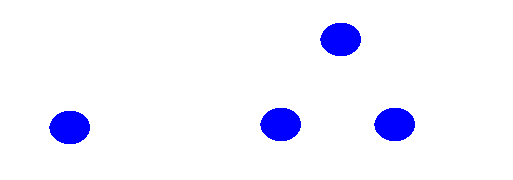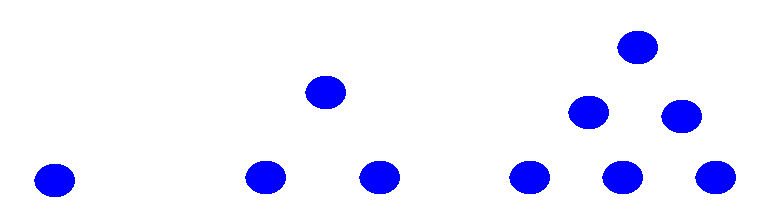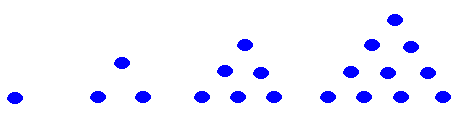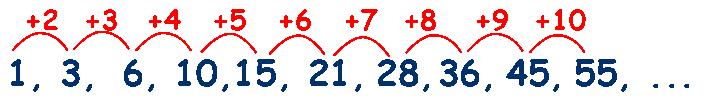Triangular Numbers

The first numbers in a triangular number sequence are therefore 1 and 3The next size of triangle you could make is 6and so on.The difference between each of the numbers increases by 1 each time.